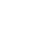Sign In## Why Albert Einstein call Time 4 Dimension ?

765 views
0

i only know that time is measure of change in mattar,if there is no mattar there is no change and there is no time.
so time had begining with time mattar and space.

why albert einstein call Time 4 Dimenstion ?

0

In Newton’s view, time was a separate variable and appeared so in the equations. But when Einstein analyzed the behavior of light in the Michelson-Morely Experiment, he found that the only way to describe the results was to write:

x^2 + y^2 + z^2 = c^2(t^2)

This formula is a conservation or “invariant” equation which means that the left hand side is always equal to the right hand side. This means that if x changes, and y and z stay the same, then t must also change. This means that space (the x-variable) and time (the t-variable) are always linked, thus making time another dimension of space.

I’m sorry if this answer is too complex, but this is not an easy topic!

0

There are a lot of different ways to think about this depending on your understanding of physics. Put simply he didn’t just call it the 4th, it IS the 4th. Take any coordinate system in space and it’s fairly easy to convert time to space just by multiplying a constant (c-the speed of light).

It’s difficult to conceive but because of relativity distance is dependent upon velocity… time dilation and length contraction means that distance and time are all relative to the observers. There is however a quantity that isn’t relative to the observer but rather constant for any two events separated by any amount of time and any distance. The invariant interval, I, would best be written for you as I=x^2+y^2+z^2-c^2t^2. Notice, t is in units of space if you multiply the constant c. The best way to view space then is as a dimension perpendicular to space. Or, if you want you could say that the entire space time continuum can be represented as a 4 dimensional matrix, with 3 spacial and one dimension of time. (In fact this is exactly what physicists do with the Minkowski space).

So… as simply as I can put it, time is called the 4th dimension because it actually is a dimension, space and time are intrinsically linked. Relativity relies on this, even if it’s difficult to conceive of.